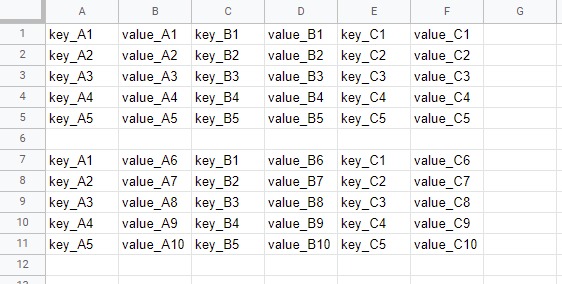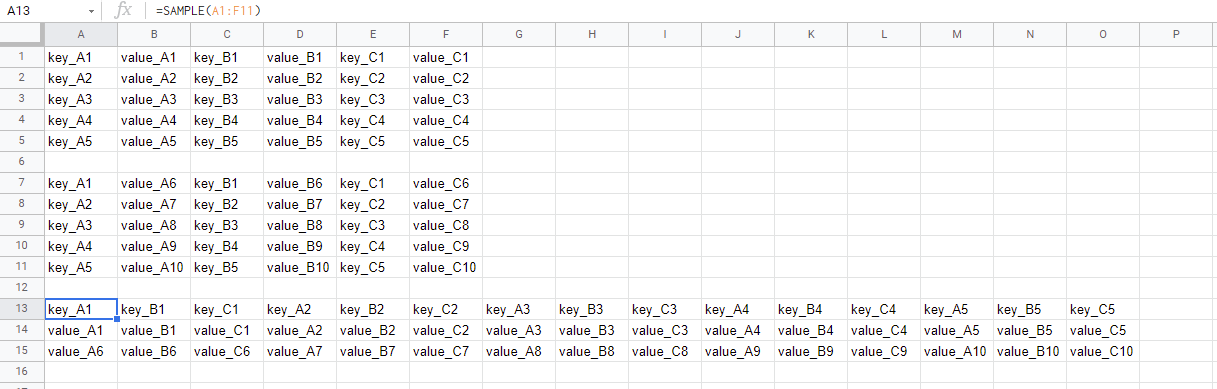Gists

This is a sample Google Apps Script for processing the values in Google Spreadsheet. In this sample situation, each row is merged using the same header title.

In this sample script, the sample input and output situations are as follows.

## Sample situation

### Input:### Output:## Sample script

In this sample script, this sample can be used as the custom function.

function SAMPLE(values) {
...new Set(
values
.map((r) => [...r])
.flatMap((r) =>
[...Array(Math.ceil(r.length / 2))].map((_) => r.splice(0, 2))
)
),
].filter(String);
const obj = values.reduce((o, r) => {
[...Array(Math.ceil(r.length / 2))].forEach((_) => {
const [k, v] = r.splice(0, 2);
if (k && headers.includes(k)) o[k] = o[k] ? [...o[k], v] : [v];
});
return o;
}, {});
const v = headers.map((e) => [e, ...obj[e]]);
return v.map((_, c) => v.map((r) => r[c]));
}

• In this sample script, the following flow is used.
1. Retrieve values from cells.